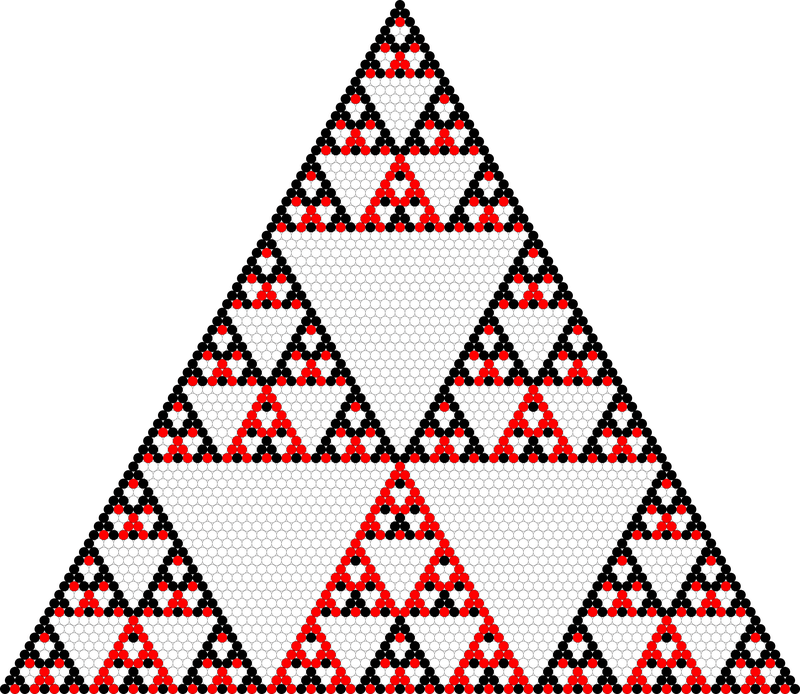# Pascal's Triangle Modulo Three

Tuesday, June 30, 2020, 11:48 PM

I think I have drawn Pascal’s triangle numerous times in this life. Once it was a eureka moment when I modulo each entry with two, resulting in a Sierpiński triangle! (remembered vividly since I drawn it on @NutSnC’s new iPad, LOL) … Today I’m experiencing that moment again, when I modulo it with three instead.Pascal’s triangle modulo three; where white, black, and red represent 0, 1, and 2 repectively1

We still get a fractal shape! Observe that the base triangle is the top 3 rows, where every dots is black except for the red center bottom. We may reconstruct the fractal triangle with iteration, by expanding each black dot with the exact base triangle, and expand each red dot with the black-red swap.

(We may also view the base as a rhombus of 3 width and 5 height, the upper 3 height is a base triangle, and the lower 2 height is just an all white triangle.)

So we may write this code for finding $\binom{n}{k} {\pmod 3}$ in $O(\log n)$ time.

table = [ [[0, 0, 0], [0, 0, 0], [0, 0, 0]],
[[1, 1, 1], [1, 2, 0], [1, 0, 0]],
[[2, 2, 2], [2, 1, 0], [2, 0, 0]], ]

def base(n):
b = 3
while b <= n:
b *= 3
return b // 3

def ternary(n, b):
while b != 0:
d, n = divmod(n, b)
b //= 3
yield d

def choose_mod3(n, k):
t = 1
for y, x in zip(ternary(n-k, base(n)), ternary(k, base(n))):
t = table[t][y][x]
return t


Don’t forget that $n$ has a binary representation of length $O(\log n)$ bits. Thus the algorithm is actually run in linear time. Which is faster that computing the whole factorial/combination using many multiplication/division.

Now (it’s time to bed) I still can’t rest my mind with the thought, what wll Pascal’s triangle with other modulo value would look like…?

1. Python script for generating the image

author